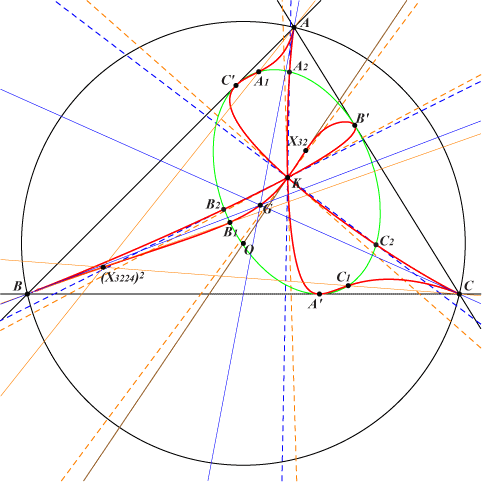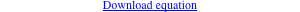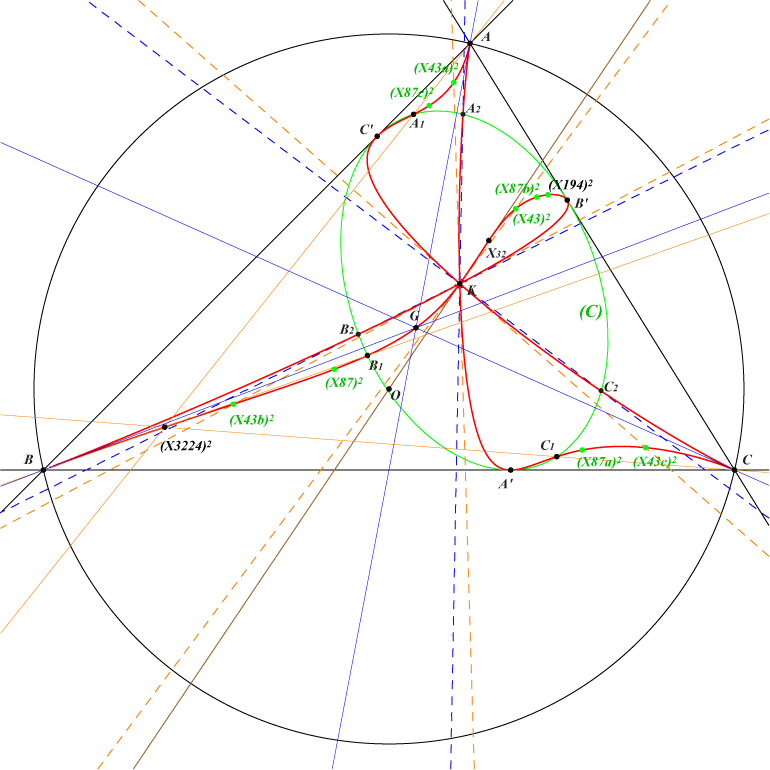too complicated to be written here. Click on the link to download a text file.X(2), X(6), X(32), X(51539), X(51540), X(53145), X(53146), X(53147), X(53148) more generally, barycentric squares of points on K102 cevians of X(32) other points belowQ184 is mentioned at the bottom of CL073. A variable line passing through X(6) meets the Grebe cubic K102 again at two points P1, P2 which are obviously isogonal conjugates, hence these points are the foci of a conic inscribed in ABC. The center of this conic lies on a nodal cubic with node X(6) and nodal tangents parallel to the asymptotes of the Jerabek hyperbola. This cubic passes through X(1), X(6), X(39), X(597), X(41369), the excenters, the infinite points of K102. This is Cax(X6) in CL047. The barycentric squares of P1, P2 are two points Q1, Q2 on Q184 which is therefore entirely inside the reference triangle ABC. Q184 is in fact the barycentric square of K102, hence it is a circum-sextic. Q184 passes through A, B, C which are cusps. The cuspidal tangents are the medians. Q184 is tangent to the sidelines of ABC at the vertices A', B', C' of the cevian triangle of X(32). These points obviously lie on (C), the inconic with perspector X(32) and center X(8265). (C) is he barycentric square of the Lemoine axis. X(6) is a quadruple point and the four (dashed orange) tangents at X(6) pass through the barycentric squares X(43)2, X(43a)2, X(43b)2, X(43c)2 of X(43) and its extraversions. These points lie on Q184. The isogonal conjugate of X(43) is X(87) hence Q184 passes through the barycentric squares X(87)2, X(87a)2, X(87b)2, X(87c)2 of X(87) and its extraversions. Remark : these triangles are perspective : • ABC and X(43a)2, X(43b)2, X(43c)2 at X(87)2, • ABC and X(87a)2, X(87b)2, X(87c)2 at X(43)2, • X(43a)2, X(43b)2, X(43c)2 and X(87a)2, X(87b)2, X(87c)2 at a point P on the Brocard axis, on the line X(43)2, X(87)2 but not on Q184. P = a^2 (a^4 b^4+6 a^4 b^2 c^2-2 a^2 b^4 c^2+a^4 c^4-2 a^2 b^2 c^4+b^4 c^4) : : , SEARCH = 0.203717834156887. P is now X(53164), also on the lines {384, 21001}, {1003, 36650}, {1184, 10997}, {1613, 3360}, {3051, 33014}, {3499, 33235}, {20965, 33022}. *** The lines passing through X(32) and A', B', C' meet (C) again at A1, B1, C1 which lie on Q184. Note that ABC and A1B1C1 are perspective at the barycentric square of X(3224), a point on Q184. These points A1, B1, C1 are the barycentric squares of the third points of K102 on the cevian lines of X(3224). Q184 and (C) have three remaining common points A2, B2, C2. They are the barycentric squares of the barycentric products of X6 and the infinite points of K102. X(53145), X(53146), X(53147), X(53148) are the barycentric squares of X(43), X(87), X(194), X(3224), now in ETC. X(51540), X(51539) are the barycentric squares of the imaginary foci X(39641), X(39642) of the Brocard ellipse. X(51540) and X(51539) lie on the line passing through {X160, X184, X571, X3135}, on the circum-conic passing through {X4, X5, X53, X311, X327, X1141, X1263, X1487, X2165, X2980} and on the cubic K1284.